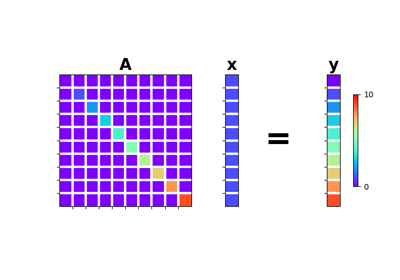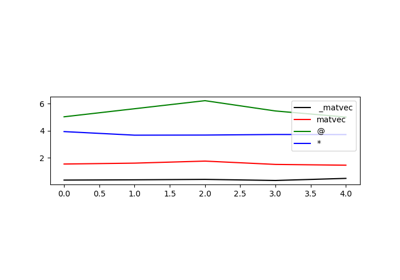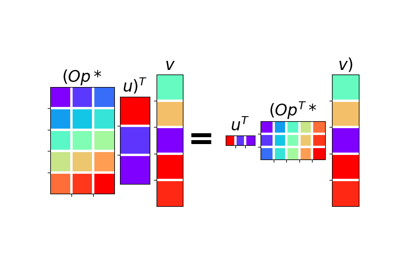# pylops.Diagonal¶

class pylops.Diagonal(diag, dims=None, dir=0, dtype='float64')[source]

Diagonal operator.

Applies element-wise multiplication of the input vector with the vector diag in forward and with its complex conjugate in adjoint mode.

This operator can also broadcast; in this case the input vector is reshaped into its dimensions dims and the element-wise multiplication with diag is perfomed on the direction dir. Note that the vector diag will need to have size equal to dims[dir].

Parameters: diag : numpy.ndarray Vector to be used for element-wise multiplication. dims : list, optional Number of samples for each dimension (None if only one dimension is available) dir : int, optional Direction along which multiplication is applied. dtype : str, optional Type of elements in input array.

Notes

Element-wise multiplication between the model $$\mathbf{x}$$ and/or data $$\mathbf{y}$$ vectors and the array $$\mathbf{d}$$ can be expressed as

$y_i = d_i x_i \quad \forall i=1,2,...,N$

This is equivalent to a matrix-vector multiplication with a matrix containing the vector $$\mathbf{d}$$ along its main diagonal.

For real-valued diag, the Diagonal operator is self-adjoint as the adjoint of a diagonal matrix is the diagonal matrix itself. For complex-valued diag, the adjoint is equivalent to the element-wise multiplication with the complex conjugate elements of diag.

Attributes: shape : tuple Operator shape explicit : bool Operator contains a matrix that can be solved explicitly (True) or not (False)

Methods

 __init__(self, diag[, dims, dir, dtype]) Initialize this LinearOperator. adjoint(self) Hermitian adjoint. cond(self, \*\*kwargs_eig) Condition number of linear operator. conj(self) Complex conjugate operator div(self, y[, niter]) Solve the linear problem $$\mathbf{y}=\mathbf{A}\mathbf{x}$$. dot(self, x) Matrix-matrix or matrix-vector multiplication. eigs(self[, neigs, symmetric, niter]) Most significant eigenvalues of linear operator. matmat(self, X) Matrix-matrix multiplication. matrix(self) Return diagonal matrix as dense numpy.ndarray matvec(self, x) Matrix-vector multiplication. rmatvec(self, x) Adjoint matrix-vector multiplication. transpose(self) Transpose this linear operator.
matrix(self)[source]

Return diagonal matrix as dense numpy.ndarray

Returns: densemat : numpy.ndarray Dense matrix.

## Examples using pylops.Diagonal¶Diagonal01. The LinearOpeator02. The Dot-Test